﻿ Structs or Records
 Structs or Records

Structs are a new feature in EXPL that allows you to create your own complex data types. Like other XPL features, structs in XPL use a combination of the best features of C and Pascal structs. Currently, none of the other versions of XPL0 support structs.

I. Programming Using Structs. Structs are user-defined, composite data types, that are sometimes called "records." The struct feature allows the programmer to define new data types, above and beyond the normal integer, real and string types built into the language. In general, a struct allows you to combine multiple variables into a single, composite data type.

1. Declaring Structs. To use a struct, you have to define it. You do this by telling the compiler that you are creating a new data type using the "type" command, followed by a name for the type. Next, you tell the compiler that the type consists of a series of "records." Finally, you provide a set of records that is basically, just a list of variables. Here is a simple example:

type TVector = record

real X,Y,Z;

end;

In keeping with the syntax of XPL, you can put a "begin" symbol at the beginning of the list of records:

type TVector = record

begin

real X,Y,Z;

end;

You can also use brackets in place of "begin" and "end."

type TVector = record

[

real X,Y,Z;

];

Once you've created a new data type, you can use it to declare variables. The variables are declared in exactly the same way you would  declare any other variable in XPL0:

TVector V,P,Loc;

int A,B,C;

The example we have been using allows you to create variables that encompass the common mathematical concept of a vector. Having this type of variable will allow you to perform vector operations in a simple, intuitive way.

Structs aren't just for mathematics. They can be used any time you want to organize and combine related pieces data. To clarify how structs are used, here is a more complex declaration that includes a string, an integer and a real value.

type TVitalStatisics = record

char Name;

int Age;

real Height;

end;

They are especially useful for implementing algorithms. For example, here is a struct-based version of a double linked list of names.

type TListItem = record

int Last,Next;

char Names;

end;

TListItem List(5000);

2. Structs In Structs. Structs can also contain other structs. In other words, in addition to the three basic data types in XPL0, structs can also contain any previously defined struct. The structs can be nested inside each other to an unlimited depth. For example.

type TPoint = record

int X,Y;

end;

type TLine = record

TPoint P1,P2;

end;

type TRect = record

Left,Right,Top,Bottom: TLine;

int Color;

end;

These example show how you can start with a simple "point" struct and build it into lines, and then rectangles that include a color property. Here is an example of how you might build a quaternion variable type:

type TVector = record

real X,Y,Z;

end;

type TQuaternion = record

TVector: V;

real W;

end;

3. Struct Names. Like all XPL0 variables, the struct-name must begin with an upper case character. The rest of the struct name can be any upper or lower case letter (A-Z, a-z), numbers (0-9), or underlines (_).

In the examples used here, the struct names begin with a "T". This is a Pascal convention that indicates that the identifier is a "type." It helps to differentiate type names from ordinary variables. The "T" is not required.

4. Declaring Struct Variables. Once you've created your own data-type, you can use it to declare variables. The syntax is identical to that of ordinary XPL0 variables. In other words, any where that you would use a "int," "char," or "real" to declare an XPL0 variable, you can declare a struct variable. Here are some examples of declaring struct variables:

TVector V;

TVector Pos,Vel,Acc;

Structs follow the same scope rules as ordinary variables. Structs must be defined before they are used. Structs defined inside subroutines are only available inside that subroutine or any nested subroutines.

5. Accessing Struct Components. To access the contents of a struct-variable, you use "dots" to specify the components of the variable. For example:

V.X:=1.23;

V.Y:=4.56;

V.Z:=7.89;

RLOut(0,V.Y);

If a struct contains another struct, you can drill down into the struct using the "." operators. For instance, in the following example, the "TRect" struct contains "TLine" components, which contain "TPoint" components, which contain "int," X and Y components:

type TPoint = record

int X,Y;

end;

type TLine = record

TPoint P1,P2;

end;

type TRect = record

Left,Right,Top,Bottom: TLine;

end;

As a result, you can selectively drill down three levels using the dot notation:

TPoint P;

TLine L;

TRect R;

int I;

L:=R.Right;                        \ Drill to the TLine level

P:=R.Left.P1;                \ Drill to the TPoint level

I:=R.Top.P2.Y;                \ Drill to the int level

6. Assigning Struct Variables. Structs can be assigned just like any other variable. When a struct is assigned to another struct, all the values are copied from the source to the destination.

TVector V1,V2;

V1.X:=1.23;

v1.Y:=4.56;

V1.Z:=7.89;

V2:=V1;      <==== V2 Now contains the same values a V1.

7. Passing Structs To Subroutines. Structs can be passed to subroutines by "value" or by "reference." .

A. Passing By Value. When they are passed by reference, the value is copied to the subroutine, so the subroutine cannot modify the original variable. Here is an example:

procedure ShowVector(V);

TVector V;

begin

Text(0,"X: "); RLOUT(0,V.X);

Text(0," Y: "); RLOUT(0,V.Y);

Text(0," Z: "); RLOUT(0,V.Z);

CRLF(0);

end;

TVector V;

begin

V.X:=1.23;

V.Y:=4.56;

V.Z:=7.89;

ShowVector(V);

end;

B. Passing By Reference. Passing by reference allows you to modify the original variable in a subroutine. To do this, you have to send the address of the struct to the subroutine so the subroutine can modify the values. This is done using the "@" operator to pass the variable address to the subroutine. Likewise, to access the struct inside a subroutine, you have to treat it as an array, using a zero index to access the content of the struct. For example:

procedure SetVector(@V,X,Y,Z);

TVector V;

real X,Y,Z;

begin

V(0).X:=X;

V(0).Y:=Y;

V(0).Z:=Z;

end;

TVector V;

begin

SetVector(@V,1.23,4.56,7.89);        \By reference

ShowVector(V);

end;

8. Returning Structs From Functions. You can also return struct values from functions. This works the same way as integer and real functions. You simply use the struct-name to specify function type. For example:

function TVector MakeVector(X,Y,Z);

real X,Y,Z;

TVector V;

begin

V.X:=X;

V.Y:=Y;

V.Z:=Z;

return V;

end;

TVector V;

begin

V:=MakeVector(1.23,4.56,7.89);

ShowVector(V);

end;

9. Struct Arrays. You can create arrays of structs in the exactly the same way you create ordinary arrays. For example:

TVector VA(5,5);

int I,J;

begin

for I:=0 to 4 do

for J:=0 to 4 do

begin

VA(I,J):=MakeVector(1.23,4.56,7.89);

end;

end;

At this point, EXPL does not allow you to define part of the struct as an array. For example, you cannot do this:

type TMyType = record

int IA(10,10);

int X;

end;

You can however, treat some items inside a struct as an array. This only applies to built-in, XPL0 data types like "int," "char," and "real." For example, you can access the individual bytes in a string that is part of a struct:

type TMyType = record

char S

int X;

end;

TMyType MT;

MS.S:="ABCDEFGHIJ";

MS.S(1);        <===== Access the second byte in the string

To do this with an integer or real struct value, you have to manually reserve the memory for the array. For example:

type TMyType = record

char S

int X;

real R;

end;

TMyType MT;

MT.X:=Reserve(10 * 4);

MT.R:=RLRes(10);

MT.X(5):=123;

MT.R(5):=1.234;

10. Aliases. Aliases allow you to create a new data type that is a stand-in for another, preexisting data type. Defining an alias is similar to creating a struct, except that you specify a data type instead of a set of records. Here is an example of creating a new name for an integer type:

type TMyType = int;

Once you've defined an alias, you can use it anywhere that you would use a standard data type. For example, the following code shows TMyType being used to declare variables and specify a return type for a function.

func TMyType Test;

TMyType X:

X:=123;

return X;

end;

The concept is not very useful when using built-in variable types such as integer and real. However the concept is useful for user-defined data types. They allow you to change a data type throughout a program without hunting for all the variables that use the type. For example, here are two similar structs:

type TVector = record

real X,Y,Z;

end;

type TVector2 = record

real X,Y,Z;

width int;

Color int;

end;

If you setup an alias for one of the structs, you can use that data type throughout the program when declaring variables:

type TMyType = TVector

TMyType V1,V2,V3;

Now, if you change your mind and want to use a different struct as the data type throughout the program, it is a simple matter to change the definition:

type TMyType = TVector2.

With that simple change, all the variables throughout the program will change too.

II. Structs and the Debugger. The Debugger also supports structs. Since structs consist of multiple components, the Debugger will display the value of part or all of the components depending where the mouse cursor is. For example, here we define a two-by-two array of vectors:

type TVector = record

real X,Y,Z;

end;

TVector VA(2,2);

 Moving the mouse curssor over the show all the components of all the array items of the struct: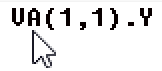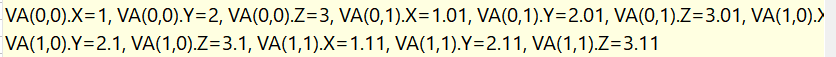Moving the cursor over the closing parenthesis of the array indices shows the value of all the components of that array item.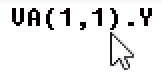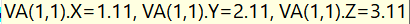Moving the mouse cursor over one of the components of the struct, shows the value for that component.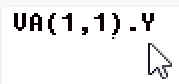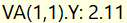Watches behave the same way, showing parts or all the struct variable's values depending on the exact string you specify.

IV. Example Programs. The easiest way to learn how to use structs is look at some example programs When you install EXPL, the setup program will load several demo programs into the installation directory that illustrate how EXPL structs work:Here is a description of each one:

Vectorlib.xpl. This file contains a 3D vector and matrix library.

ConnicalTest.xpl. This file contains test routines that systematically tests every struct operation that the compiler has to handle.

StructsAllTests.xpl This file also rigorously tests the compiler's handling of structs but focuses on more complicated constructs.

MatrixTest.xpl. This program allows you display and manipulate a 3D cube.using Vector and Matrix operations. It uses structs to simplify the operations.

III. Technical Details.

1. Syntax Diagram. The following image is a syntax diagram for structs in EXPL: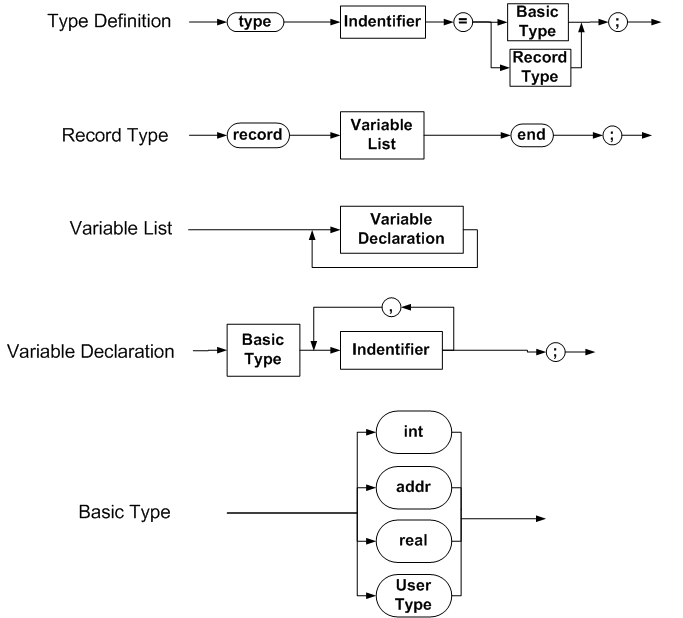2. Returning structs. In previous versions of XPL0, functions return values by storing them in variable address Global-Zero. To make sure that "real" values will fit in Global-Zero, eight bytes are reserved for Global-Zero.

Since structs can be of any size, structs are handled differently. If a "return" command is executed with a struct as the argument, the value of the struct is stored at the top of the heap. The address of the top of the heap is stored in Global-Zero.

When the "return" command is executed, the program returns from the subroutine. If the subroutines is being used as a factor, the code retrieves the heap-address from the Global-Zero and then pushes the struct value onto the stack. Even though returning from a subroutine decrements the Heap Pointer, the data on the heap is not changed. That's because the data is removed immediately before any other instructions can be executed.

3. I2L Opcodes. There are eleven new I2L Opcodes that correspond to similar non-struct versions. There are four loads, four stores and three handling stack/heap operations

LSX  = \$61, Load Struct Indexed

LIRS = \$62, Load Indexed Real Struct

LIIS = \$63, Load Indexed Integer Struct

LIBS = \$64, Load Indexed Byte Struct

Store Instructions

SIRS = \$65, Store Indexed Real Struct

SIIS = \$66, Store Indexed Integer Struct

SIBS = \$67, Store Indexed Byte Struct

SSX  = \$68, Store Structure Indexed

Stack and Heap Instructions

PISA = \$69, Push Indexed Struct Address

PINS = \$6A, Push indirect struct

SSHP = \$6B, Store Structure On top of Heap

4. Longer Variable Names. Because structs can have significantly longer names, the number of significant characters in an identifier has been increased from 16 to 64.

5. Compiler Struct Arrays. The Compiler stores struct data in six different tables. They are simple, one-dimensional arrays.

A. UserTypeNames. This item is an array of strings and it stores the type-name of each struct.

B. SubitemCount. This item is an array of integers and it stores the number of sub-items or components of a struct.

C. SubitemIndices. This item is an array of integer and it is an index to the start of the corresponding sub-items in the sub-item arrays.

D. SubitemName, This item is an array of strings and it stores the variable name of each struct's sub-item.

E. SubitemType. This item is an array of integers and it stores the variable type of each sub-item

F. SubUserIndex. This item is an array of integers. If the sub-item is a struct data-type, this item is a pointer to the struct in the table.

As an example, here is what the tables would look like for the following set of structures:

type MyType1 = record

real X,Y,Z;

end;

type MyType2 = record

real A,B,C;

MyType1 D;

end;

type MyType3 = record

int I,J;

end;

type MyAlias = MyType2;

 Inx UserTypeNames SubitemCount SubitemIndices Inx SubitemName SubitemType SubUserIndex 0 "MyType1" 3 0 0 "X" Real NA 1 "Y" Real NA 2 "Z" Real NA 1 "MyType2" 4 3 3 "A" Real NA 4 "B" Real NA 5 "C" Real NA 6 "D" UserType 0 (MyType1) 2 "MyType3" 2 7 7 "I" Integer NA 8 "J" Integer NA 3 "MyAlias" 1 9 9 Empty UserType 1 4 "MyTypeN" ... ... ... ... ... ...

As an example of how structs can simplify code, here is the same set of six tables converted to just two tables using structs

type TStructInfo = record

char Name;

int Count, Index;

end;

type TSubItem = record

char SubName;

int SubType,StructIndex;

end;

TStructInfo Structs(1000);

TSubItem SubItems(10,000);

6. Compiler Modifications Summary. In order for XPL) to handle structs, the Compiler had to be modified in four different places:: IDFAC, SPECFAC, ASSIGN TO and RETURN. Here is a description of the modifications:

+ Assuming the following variable declarations

type TVector = record

real X,Y,Z;

end;

TVector V;

TVector VA(10);

TVector V2D(10,10);

+ And a Push Order of  1) Base, 2) Size, 3) Index 4) Offset

i. IDFAC

a. V - Load onto stack the content of the struct. Repeat "LOD" on stack

using Level/Offset number of words = struct size

b. V.Y - Float load "FLOD" of value at a specific Level/Offset.

c. VA(I) - Push Base, Size, Index, Offset and use LSX

d. VA(I).Y - Push Base, Size, Index, Offset and use LIRS

e. V2D(I,J) - Push Base, Size, Index use PINS for N-1 Indices, then Push Offset, use LSX

f. V2D(I,J).Y - Push Base, Size, Index use PINS for N-1 Indices, then Push Offset, use LIRS, LIIS, or LIBS

g. Struct Return Function.

ii. SPECFAC - @

a. @V - Ues ADDR to get variables base address.

b. @V.Y - Use ADR Level and Offset to address

c. @VA(I) - Push base, size, index, Offset then use PISA.

d. @VA(I).Y

a. Push Base, Size, Index use PINS for N-1 Indices.

b. Push size, index, Offset then use PISA.

c. Push offset then use ADD to address

f. @V2D(I,J)

a. Push Base, Size, Index use PINS for N-1 Indices.

b. Push size, index, Offset then use PISA.

6. @V2D(I,J).Y

a. Push Base, Size, Index use PINS for N-1 Indices.

b. Push size, index, Offset then use PISA.

c. Push offset then use ADD to address

iii. ASSIGN TO

a. V - Repeat STO(Level,Offset + I), number times = (Sizeof / IntSize)

b. V.Y - STO(Level,Offset + Struct Component Offset)

c. VA(I) -

a. Push Base, Size, Index, Offset and use PISA to form addres,

b. load right side of assignment

c. Push size, then store using SSX.

d. VA(I).Y -

a. Push Base, Size, Index, Offset and use PISA to form addres,

b. load right side of assignment

c. Store using SIRS.

e. V2D(I,J) -

a. Push Base, Size, Index use PINS for N-1 Indices,

b. Push Base, Size, Index, Offset and use PISA to form addres,

c. Load right side of assignment through BOOLEXP

d. Store using SIRS.

f. V2D(I,J).Y -

a. Push Base, Size, Index use PINS for N-1 Indices

b. then Push Offset, use SIRS, SIIS, or SIBS opcode

IV. RETURN

+ V or V(I) or V(I,J)

a. Call BOOLEXP to struct on the stack.

b. Push Size on Stack.

c. Put struct at top of heap using SSHP opcode